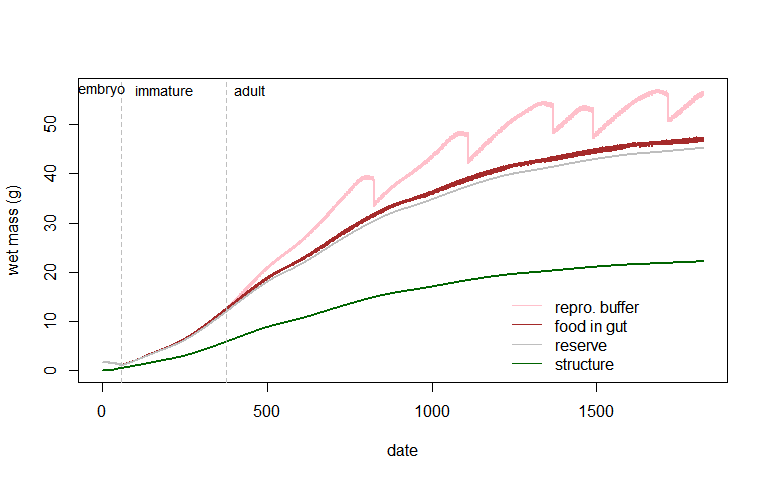# Overview

This vignette is a tutorial for the NicheMapR ectotherm model (Kearney and Porter, 2019). It only considers the steady state heat and (partial) water budget model. For details on the implementation of the Dynamic Energy Budget model (for simulating growth, development and reproduction as well as full water budgets), see the vignette Introduction to Dynamic Energy Budget models in NicheMapR.

The NicheMapR ectotherm model is run with the function ectotherm. It includes a range of morphological, physiological and behavioural options to capture how organisms exchange heat and water with their environments, and to compute the consequences of this for body temperature, activity constraints and different aspects of the mass budget. It is designed to take the output of the NicheMapR microclimate model (Kearney and Porter, 2017) as the driving environmental inputs, but you could construct custom versions of these if desired.

# Basic operation

As with the Introduction to the NicheMapR microclimate model, this first example involves the most basic case of running the model to produce 12 days of output, one for each month of the year (but the model can be run from any outputs from the various microclimate model functions). Try running the model with the default inputs for a location of your choice, e.g.:

library(NicheMapR)
longlat <- c(146.77, -19.29) # Townsville, northern Australia
micro <- micro_global(loc = longlat)
ecto <- ectotherm() # uses default settings (the Eastern Water Skink)

The results are returned as a list of tables, here called ecto. The main ones to focus on for now are

• environ The body temperature, activity and environments experienced by the animal
• enbal The components of the computed heat budget
• masbal The components of the computed mass budget

# Output table environ

The output table environ looks like this (some columns of the first 13 hrs are shown):

TIME TC SHADE SOLAR DEP ACT TA TSUB TSKY VEL RELHUM ZEN ABSAN
0 28.97 0 0.00 -2.5 0 28.77 28.77 28.77 0.01 99.00 90.00 0.85
1 28.44 0 0.00 -2.5 0 28.25 28.25 28.25 0.01 99.00 90.00 0.85
2 27.98 0 0.00 -2.5 0 27.80 27.80 27.80 0.01 99.00 90.00 0.85
3 27.60 0 0.00 -2.5 0 27.42 27.42 27.42 0.01 99.00 90.00 0.85
4 27.26 0 0.00 -2.5 0 27.09 27.09 27.09 0.01 99.00 90.00 0.85
5 26.95 0 0.00 -2.5 0 26.78 26.78 26.78 0.01 99.00 90.00 0.85
6 29.68 0 55.23 0.0 2 24.88 25.50 16.48 0.12 78.54 83.13 0.85
7 29.76 33 95.16 0.0 2 27.18 29.94 18.45 0.19 68.52 69.68 0.85
8 29.93 54 78.52 0.0 2 28.76 32.44 20.43 0.27 62.89 55.98 0.85
9 29.74 72 40.92 0.0 2 29.34 33.18 22.57 0.34 61.87 42.12 0.85
10 29.72 84 16.21 0.0 2 29.67 32.48 24.50 0.41 61.50 28.17 0.85
11 30.23 90 7.08 0.0 2 30.24 32.82 25.95 0.49 54.38 14.20 0.85
12 31.07 90 7.33 0.0 2 31.10 33.42 26.82 0.64 54.38 1.93 0.85
PTCOND POSTURE
0 0
0 0
0 0
0 0
0 0
0 0
0 0
0 0
0 0
0 0
0 0
0 0
0 0

and includes the following variables (among others):

• TIME - time of day (hours)
• TC - body temperature (°C)
• SHADE - shade selected (%)
• SOLAR - solar radiation (W/m2) at animal location
• DEP - depth below ground (cm)
• ACT - activity state (0=inactive, 1=basking, 2=foraging)
• TA - air temperature (°C) at animal location
• TSUB - substrate temperature (°C) at animal location
• TSKY - overhead (sky) temperature (°C) at animal location
• VEL - wind speed (m/s) at animal location
• RELHUM - relative humidity (%) at animal location
• ZEN - zenith angle of sun (degrees, where 90° = below the horizon)
• ABSAN - solar absorptivity (fractional)
• PCOND - proportion of animal’s surface in contact with ground (fractional)
• POSTURE - postural orientation (1=perpendicular to sun, 2=parallel, 0=in-between)

The model has considered, for each hour of the day, where the organism could be in its habitat conditional on the behavioural options set. The default simulation is for the Eastern Water Skink, Eulamprus quoyii, which is diurnal (diurn = 1, nocturn = 0, crepus = 0). So, for the nighttime and twilight hours, the animal is assumed to be underground (burrow = 1).

The algorithm choosing the depth at which to put the animal starts at the shallowest depth allowed (here node 2 which is 2.5 cm, but it could be node 1 which is the surface) and moves deeper only if it would go below the stated critical thermal minimum CT_min (6 °C by default) or if it would be more than half way between the maximum foraging temperature T_F_max (34 °C by default) and the critical thermal maximum CT_max (40 °C by default).

Note how the wind speed is set to an arbitrarily low value when then animal is sheltering under ground, and the air, substrate and ‘sky’ temperatures are equal to the soil temperature at the chosen depth.

When the sun rises (ZEN < 90°, at 6 am in in this case) the model tries placing the animal on the surface in the open, as long as the animal’s body temperature is above the emergence temperature T_RB_min (i.e. retreat to basking transition, which is 17.5 °C by default). If the resulting computed body temperature is above the minimum basking threshold T_B_min (also 17.5 °C by default), that becomes the selected environment and the activity state ACT is reported as 1.

If the body temperature is above the minimum foraging temperature T_F_min, then activity state is set to 2. If the animal’s predicted temperature is above the preferred temperature T_pref (30 °C by default), then the animal changes posture to be parallel to the sun’s rays and thereby minimise solar heat gain. If this prevents the T_pref from being exceeded then the animal is recorded to be in this posture.

If the postural adjustment fails, then shade seeking is invoked if allowed (shade_seek = 1), which is what has happened in this example at 7 am. The shade is set to jump up in 3% intervals (delta_shade = 3), and 33% was sufficient in this case. As the day moves on, the animal in this example can remain active near its preferred body temperature but only by moving into deeper shade, to the maximum level of 90% in this case (vector maxshades which, by default, comes from the microclimate model settings).

Once the maximum shade is reached, the animal’s preferred temperature is allowed to rise in 0.5 degree increments until the maximum foraging temperature is reached T_F_max, upon which the animal is forced to become inactive.

The animal may also change its posture when cold to point normal to the sun (postur = 1) and potentially increase the contact with the substrate (pcond will increase by 20%) if the ground is warmer than the body. These postural adjustments aren’t necessary in this particular simulation, but can be important under cooler climates.

# Output table enbal

The output table enbal looks like this:

DOY YEAR DAY TIME QSOL QIRIN QMET QEVAP QIROUT QCONV QCOND ENB NTRY
15 1 1 0 0.00 4.74 0.02 0.00 4.75 0.01 0 0 1
15 1 1 1 0.00 4.71 0.02 0.00 4.72 0.01 0 0 1
15 1 1 2 0.00 4.68 0.02 0.00 4.69 0.01 0 0 1
15 1 1 3 0.00 4.66 0.02 0.00 4.67 0.01 0 0 1
15 1 1 4 0.00 4.64 0.02 0.00 4.65 0.01 0 0 1
15 1 1 5 0.00 4.62 0.01 0.00 4.63 0.01 0 0 1
15 1 1 6 1.27 4.28 0.02 0.01 4.80 0.76 0 0 1
15 1 1 7 0.79 4.47 0.02 0.01 4.80 0.48 0 0 13
15 1 1 8 0.43 4.61 0.02 0.01 4.81 0.23 0 0 20
15 1 1 9 0.17 4.70 0.02 0.01 4.80 0.08 0 0 26
15 1 1 10 0.06 4.73 0.02 0.01 4.80 0.00 0 0 30
15 1 1 11 0.02 4.79 0.02 0.01 4.83 -0.02 0 0 33
15 1 1 12 0.02 4.83 0.02 0.01 4.89 -0.02 0 0 35

It quantifies the different pathways of exchange in each hour of the simulation:

• DOY - day of year
• YEAR - year of simulation
• DAY - day of simulation
• TIME - time of day (hours)
• TC - body temperature (°C)
• QSOL - solar radiation absorbed (W)
• QIRIN - infrared radiation absorbed (W)
• QMET - metabolic heat production (W)
• QEVAP - evaporative heat loss (W)
• QIROUT - infrared radiation lost (W)
• QCONV - heat lost by convection (W)
• QCOND - heat lost by conduction (W)
• ENB - energy balance (°C)
• NTRY - iterations required for solution to heat balance equation

where the complete heat budget is

$ENB = QSOL + QIRIN + QMET - QEVAP - QIROUT - QCONV - QCOND$

QIRIN, QIROUT, QSOL and QMET will always be positive, but QEVAP, QCONV and QCOND may be positive or negative. For example in this case, QCOND is near zero in the burrow, positive (i.e. causing cooling) in the morning, and becomes negative (causing heating) by 8 am. Note that more iterations of the model, NTRY, are required when the animal is out of its burrow, due to the model repeatedly trying different options (e.g. increasing shade, increasing the target temperature, etc.) until it finds a solution.

# Output table masbal

Finally, the table masbal includes the following outputs (among others):

DOY YEAR DAY TIME O2_ml H2OResp_g H2OCut_g H2OEye_g
15 1 1 0 3.136736 -0.0000449 0.0000943 0.0000000
15 1 1 1 2.995560 -0.0000426 0.0000896 0.0000000
15 1 1 2 2.877166 -0.0000407 0.0000856 0.0000000
15 1 1 3 2.781677 -0.0000391 0.0000825 0.0000000
15 1 1 4 2.701172 -0.0000377 0.0000798 0.0000000
15 1 1 5 2.629257 -0.0000364 0.0000775 0.0000000
15 1 1 6 3.337072 0.0010420 0.0064634 0.0019396
15 1 1 7 3.360834 0.0010633 0.0077978 0.0023401
15 1 1 8 3.412029 0.0010974 0.0088309 0.0026501
15 1 1 9 3.355236 0.0010193 0.0090396 0.0027127
15 1 1 10 3.349587 0.0009917 0.0089068 0.0026729
15 1 1 11 3.501865 0.0012782 0.0123643 0.0037104
• DOY - day of year
• YEAR - year of simulation
• DAY - day of simulation
• TIME - time of day (hours)
• O2_ml - oxygen consumption rate (ml/h)
• H2OResp_g - respiratory water loss (g/h)
• H2OCut_g - cutaneous water loss (g/h)
• H2OEye_g - ocular water loss (g/h)

There are a number of other columns in this output but they are of value zero because we are not running the Dynamic Energy Budget model (i.e. DEB = 0 by default). We are instead obtaining the oxygen consumption rate (ml/h) by an allometric function based on Eq. 2 from Andrews & Pough 1985,

$$V_{O_2} = M_1 M^{M_2} 10^{M_3 T_b}$$

where $$M$$ is the mass in grams, $$T_b$$ is the body temperature, and $$M_1$$, $$M_2$$ and $$M_3$$ are constants which can be user-defined. From this, in conjunction with the oxygen extraction efficiency F_O2 and the difference in temperature between expired and inspired air delta_air (0.1 °C by default), the respiratory water loss H2OResp_g can be computed. Note that it is negative in the burrow, i.e. a tiny amount of water is gained from breathing, due to the air being highly saturated.

From the percentage of the skin that is ‘wet’ pct_wet one can estimate the cutaneous water loss H2O_cut. The pct_wet parameter represents the % surface area that would act as free water surface if all the little pores letting water through the skin were collected together in one place.

Finally, the parameter pct_eyes is the % of the total surface area taken up by the eyes when open, and is used to compute ocular water loss when the animal is active (this can be a substantial part of the water loss).

If you are interested in the other quantities available in the masbal output, you will need to develop a DEB model for your species (see the Dynamic Energy Budget lab, particularly the Add-my-pet Portal, for details, as well as the vignette Introduction to Dynamic Energy Budget models in NicheMapR which explains the debout output table).

# Plotting some output

Now let’s take broader look at the simulation outcome, by plotting the results. We will this time be explicit about the parameter values as follows (see the help for the ectotherm model by typing ?ectotherm in the R console to get more details on the options for these and other parameters):

Ww_g <- 40        # wet weight of animal (g)
pct_wet <- 0.2    # % of surface area acting as a free-water exchanger
alpha_min <-0.85  # minimum solar absorbtivity (dec %)
alpha_max <- 0.85 # maximum solar absorbtivity (dec %)
shape <- 3        # lizard shape
T_RB_min <- 17.5  # min Tb at which they will attempt to leave retreat
T_B_min <- 17.5   # min Tb at which leaves retreat to bask
T_F_min <- 24     # minimum Tb at which activity occurs
T_F_max <- 34     # maximum Tb at which activity occurs
T_pref <- 30      # preferred Tb (will try and regulate to this)
CT_max <- 40      # critical thermal minimum (affects choice of retreat)
CT_min <- 6       # critical thermal maximum (affects choice of retreat)
mindepth <- 2     # min depth (node, 1-10) allowed
maxdepth <- 10    # max depth (node, 1-10) allowed
shade_seek <- 1   # shade seeking?
burrow <- 1       # can it burrow?
climb <- 0        # can it climb to thermoregulate?
nocturn <- 0      # nocturnal activity
crepus <- 0       # crepuscular activity
diurn <- 1        # diurnal activity
minshades <- rep(0, 12)   # min available shade?
maxshades <- micro$MAXSHADES # max available shade? Run the ectotherm model, retrieve the output and create some mock dates. ecto <- ectotherm(Ww_g = Ww_g, alpha_max = alpha_max, alpha_min = alpha_min, shape = shape, pct_wet = pct_wet, T_F_max = T_F_max, T_F_min = T_F_min, T_B_min = T_B_min, T_RB_min = T_RB_min, CT_max = CT_max, CT_min = CT_min, T_pref = T_pref, mindepth = mindepth, maxdepth = maxdepth, shade_seek = shade_seek, burrow = burrow, climb = climb, minshades = minshades, nocturn = nocturn, diurn = diurn, crepus = crepus, maxshades = maxshades) # retrieve output environ <- as.data.frame(ecto$environ) # behaviour, Tb and environment
enbal <- as.data.frame(ecto$enbal) # heat balance outputs masbal <- as.data.frame(ecto$masbal) # mass balance outputs
metout <- as.data.frame(micro$metout) # above ground microclimate environ <- cbind(environ,metout$SOLR) # add solar radiation for activity window plots
colnames(environ)[ncol(environ)] <- "Solar"

# append dates
days <- rep(seq(1,12),24)
days <- days[order(days)]
dates <- days+metout$TIME/60/24-1 # dates for hourly output dates2 <- seq(1,12,1) # dates for daily output metout <- cbind(dates,metout) environ <- cbind(dates,environ) masbal <- cbind(dates,masbal) enbal <- cbind(dates,enbal) Plot the body temperature, shade, activity and depth selected through time. with(environ, plot(TC ~ dates, ylab = "", xlab="month of year", col = 'black', xlim = c(-0.25, 12), ylim = c(-20, 40), type = "l", yaxt = 'n')) with(environ, points(ACT * 2 + 7 ~ dates, type = "p", pch = 16, col = "orange")) with(environ, points(SHADE / 10 - 6 ~ dates, type = "l", col = "dark green")) with(environ, points(DEP - 10 ~ dates, type = "l", col = "brown")) abline(ecto$T_F_min, 0, lty = 2, col = 'blue')
abline(ecto$T_F_max, 0, lty = 2, col = 'red') ytick<-seq(15, 40, by=5) axis(side=2, at=ytick, labels = TRUE) mtext(text = c('A', 'B', 'I'), side = 2, line = 1, at = c(11, 9, 7)) ytick<-seq(-6, 4, by=2) axis(side=2, at=ytick, labels = FALSE) mtext(text = seq(0, 100, 20), side = 2, line = 1, at = seq(-6, 4, 2), las = 2) ytick<-seq(-20, -10, by=2) axis(side=2, at=ytick, labels = FALSE) mtext(text = rev(seq(0, 100, 20)), side = 2, line = 1, at = seq(-20, -10, 2), las = 2) abline(h = -10, lty = 2, col = 'grey') mtext(text = c('body temperature (°C)', 'activity', 'shade (%)', 'depth (cm)'), side = 2, line = 2.5, at = c(30, 9, 0, -15)) text(0.1, c(ecto$T_F_max + 1, ecto\$T_F_min + 1), c('T_F_max', 'T_F_min'), col = c('red', 'blue'), cex = 0.75)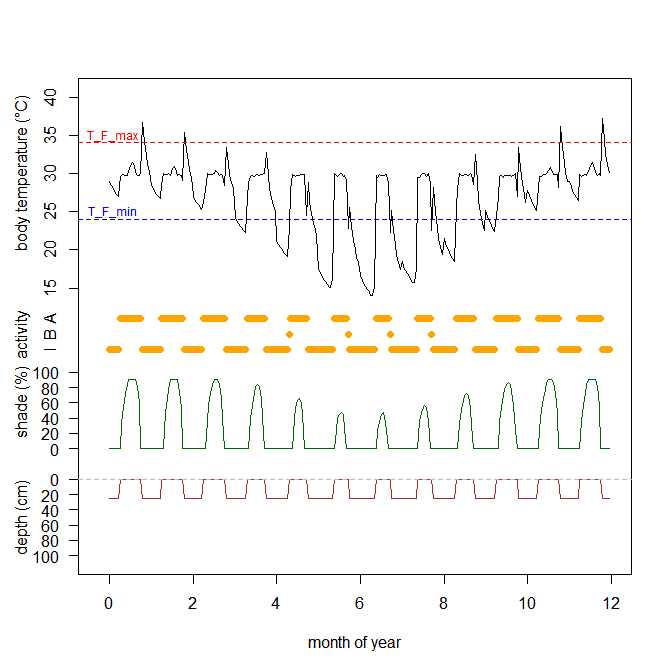Body temperature, depth, shade and activity of for the lizard Eulamprus quoyii with shade options ranging from 0% to 90%

In Figure 1 you can see the daily cycles, for each month, of the body temperature (black), % shade selected (green, divided by 10 for plotting), activity level in orange (multiplied by 5 for plotting, so 5 = basking, 10 = active) and depth in brown (m). Also superimposed are the foraging thresholds T_F_min (blue dashed line) and T_F_max (red dashed line), and the preferred or target temperature T_pref (orange dashed line).

Note how this lizard is active every day, but requires shade for this, and how body temperature jumps up on some evenings when it goes into its burrow (remember when inactive the body temperature may rise to a maximum of half way between T_F_max and CT_max before the animal moves deeper into the burrow).

# seasonal activity plot (dark blue = night, light blue = basking, orange = foraging)
forage <- subset(environ, ACT == 2) # get foraging hours
bask <- subset(environ, ACT == 1) # get basking hours
night <- subset(environ, Solar == 0) # get night hours
with(night, plot(TIME ~ DOY, ylab = "Hour of Day", xlab = "Day of Year", pch = 15, cex = 2,
col = 'dark blue')) # nighttime hours
with(forage, points(TIME ~ DOY, pch = 15, cex = 2, col = 'orange')) # foraging Tbs
with(bask, points(TIME ~ DOY, pch = 15, cex = 2, col = 'light blue')) # basking Tbs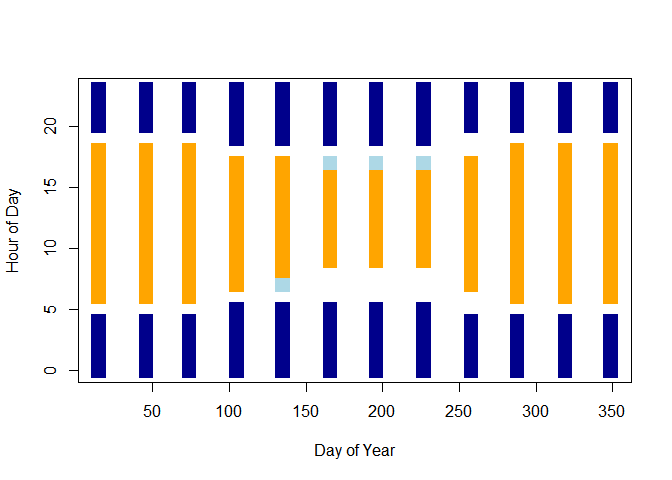Annual activity window for the lizard Eulamprus quoyii with shade options ranging from 0% to 90%

In Figure 2 you can see the annual activity window, with dark blue representing the nighttime hours, light blue the basking hours and orange the foraging hours. Activity is possible for this lizard at this tropical location throughout they year.

Explore what changing the thermal thresholds CT_min, T_RB_min, T_B_min, T_F_min, T_F_max and CT_max does to these plots, as well as variation in available shade maxshades and burrow depths mindepth and maxdepth. Here is an example of the effect of reducing maximum shade in all months from 90% to 10%: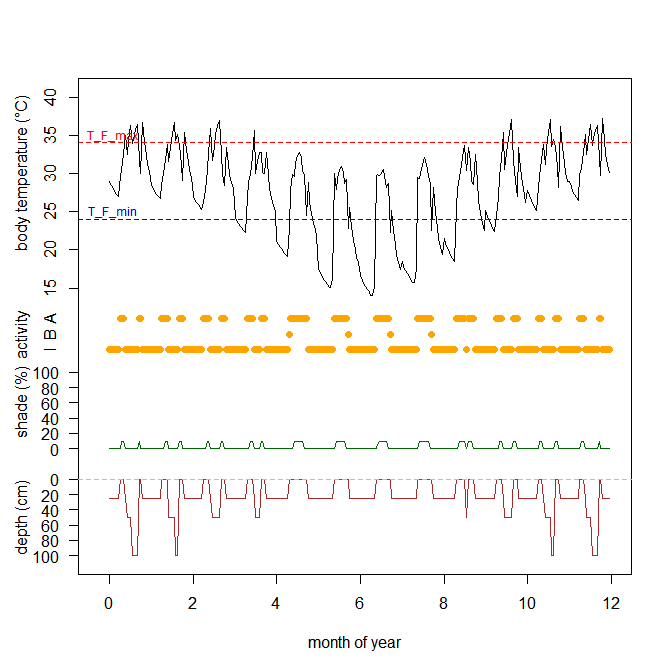Body temperature, depth, shade and activity of for the lizard Eulamprus quoyii with shade options ranging from 0% to 10%

forage<-subset(environ,ACT==2)
bask<-subset(environ,ACT==1)
night<-subset(environ,Solar==0)
day<-subset(environ,Solar==0)
with(night,plot(TIME ~ DOY,ylab="Hour of Day",xlab="Day of Year",pch=15,cex=2,col=
'dark blue'))
# nighttime hours
with(forage,points(TIME~DOY,pch=15,cex=2,col='orange')) # foraging Tbs
with(bask,points(TIME~DOY,pch=15,cex=2,col='light blue')) # basking Tbs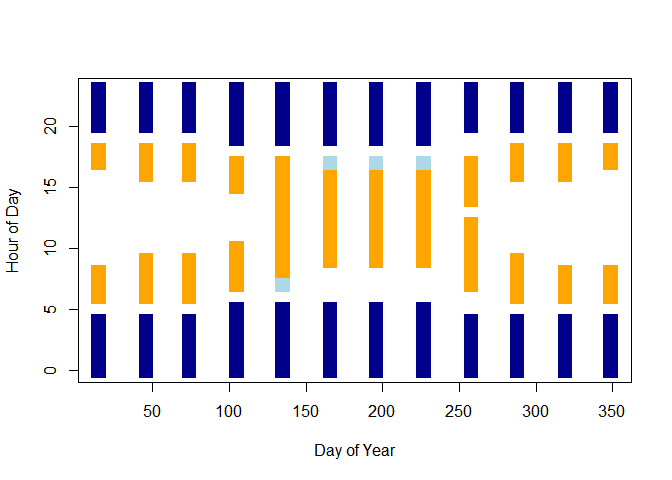Annual activity window for the lizard Eulamprus quoyii with shade options ranging from 0% to 10%

# Discussion

The capacity to compute body temperature, metabolic rates, activity times and some aspects of the water budget under realistic microclimates allows many inferences to be made about the ability of different types of animals to live in different climates and habitats. In some cases such processes may be limiting factors of the distribution. For example, Kearney and Porter (2004) found that activity time was likely to be a limiting factor for a nocturnal lizard in southern Australia. More often, these constraints act to limit the overall energy and water budget in a more subtle manner.

The preliminary computations made in this tutorial can provide the starting point for an energy and mass budget analysis that could ultimately allow you to predict reproductive success and survival rates, the ‘vital rates’ that link individuals to populations.

One of the most powerful ways of achieving a full mass balance and life cycle trajectory is by using the Dynamic Energy Budget (DEB) theory and its associated models (Kooijman, 2010). DEB theory integrated into the NicheMapR package as described further in the vignette Introduction to Dynamic Energy Budget models in NicheMapR.

Below is an example of the sort of calculation that can be made with the integration of DEB theory and the ectotherm model - a simulation of the Australian Water Skink, Eulamprus quoyii growing at Townsville Australia (see Schwarzkopf et al. 2016). This example is explained further in the DEB vignette just mentioned.